# GSEB Solutions Class 12 Maths Chapter 7 Integrals Ex 7.10

Gujarat Board GSEB Textbook Solutions Class 12 Maths Chapter 7 Integrals Ex 7.10 Textbook Questions and Answers.

## Gujarat Board Textbook Solutions Class 11 Maths Chapter 7 Integrals Ex 7.10

Evaluate the following integrals:
Question 1.
$$\int_{0}^{1}$$ $$\frac{x}{x^{2}+1}$$ dx
Solution:
Let I = $$\int_{0}^{1}$$ $$\frac{x}{x^{2}+1}$$ dx.
Put x2 + 1 = t, 2x dx = dt.
When x = 1, t = 2 and when x = 0, t = 1.
∴ I = $$\int_{2}^{1}$$ $$\frac{dt}{t}$$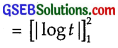= log 2.Question 2.
$$\int_{0}^{\frac{\pi}{2}}$$ $$\sqrt{sinϕ}$$cos5ϕdϕ
Solution:
Let I = $$\int_{0}^{\frac{\pi}{2}}$$ $$\sqrt{sinϕ}$$cos5ϕdϕ
= $$\int_{0}^{\frac{\pi}{2}}$$ $$\sqrt{sinϕ}$$cos4ϕcosϕdϕ
Put sinϕ = t so that cosϕ dϕ = dt.
When ϕ = 0, t = 0 and when ϕ = $$\frac{π}{2}$$, t = 1.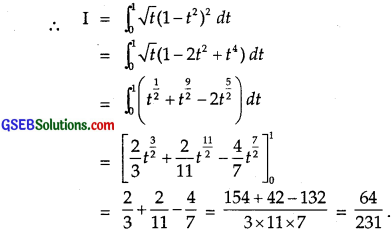Question 3.
$$\int_{0}^{1}$$ sin-1($$\frac{2 x}{1+x^{2}}$$)dx
Solution: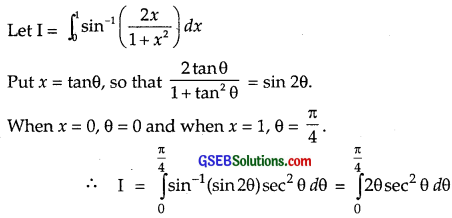Integrating by parts, taking θ as first function,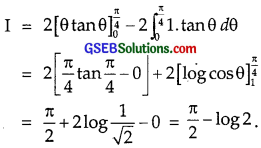Question 4.
$$\int_{0}^{2}$$x$$\sqrt{x+2}$$ dx
Solution:
Let I = $$\int_{0}^{2}$$x$$\sqrt{x+2}$$ dx
Put x + 2 = t2 so that dx = 2t dt.
When x = 0, t = $$\sqrt{2}$$ and when x = 2, t2 = 4 ⇒ t = 2.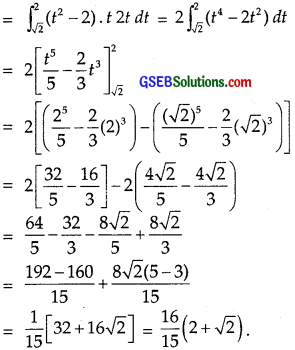Question 5.
$$\int_{0}^{\frac{\pi}{2}}$$ $$\frac{\sin x}{1+\cos ^{2} x}$$ dx
Solution:
Let I = $$\int_{0}^{\frac{\pi}{2}}$$ $$\frac{\sin x}{1+\cos ^{2} x}$$ dx
Put cos x = t so that – sin x dx = dt.
When x = 0, t = cos 0 = 1 and when x = $$\frac{π}{2}$$, t = cos $$\frac{π}{2}$$ = 0.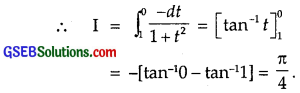Question 6.
$$\int_{0}^{2}$$ $$\frac{d x}{x+4-x^{2}}$$
Solution: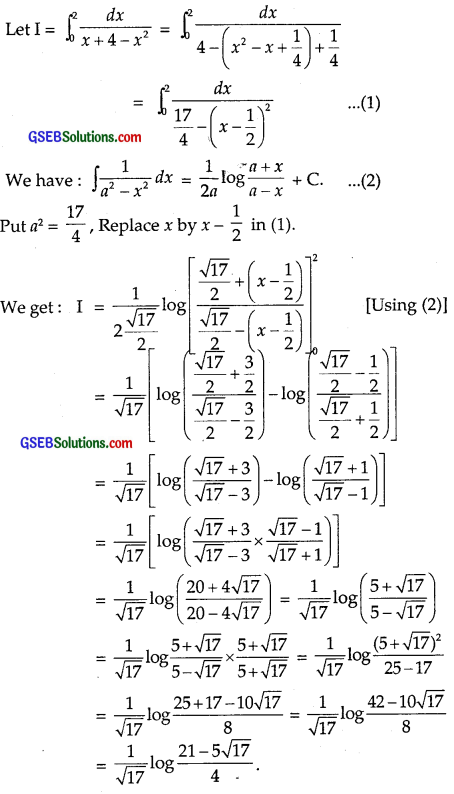Question 7.
$$\int_{-1}^{1}$$ $$\frac{d x}{x^{2}+2 x+5}$$
Solution:
Let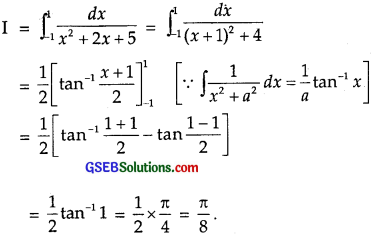Question 8.
$$\int_{1}^{2}$$ ($$\frac{1}{x}$$ – $$\frac{1}{2 x^{2}}$$ e2x dx
Solution: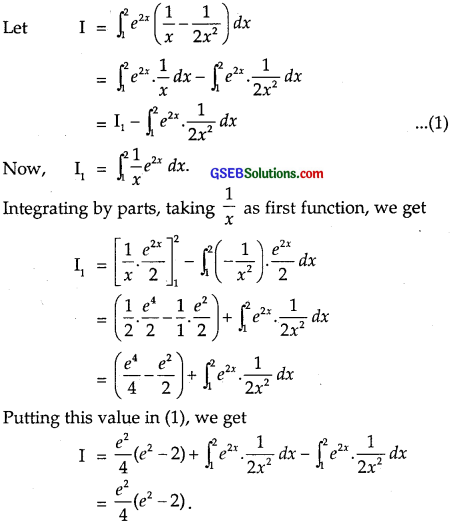Choose the correct answers in questions 9 and 10:
Question 9.
The value of the integral $$\int_{\frac{1}{3}}^{1} \frac{\left(x-x^{3}\right)^{\frac{1}{3}}}{x^{4}}$$ dx is
(A) 6
(B) 0
(C) 3
(4) 4
Solution: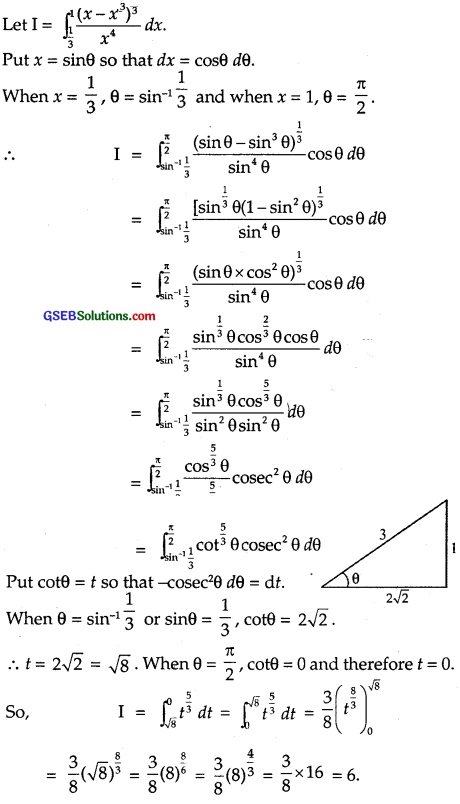∴ Part (A) is the correct answer.Question 10.
If f(x) = $$\int_{0}^{x}$$ tsint dt, then f'(x) is
(A) cos x + x sin x
(B) x sin x
(C) x cos x
(D) sin x + x cos x
Solution:
R.H.S. = f(x) = $$\int_{0}^{x}$$ tsint dt
Integrating, by parts taking t as first function, we get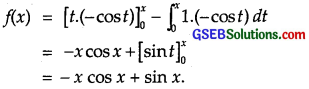∴ f'(x) = – [1.cos x – x sin x] + cos x = x sin x.
∴ Part (B) is the correct answer.